# C++ Program to check number positive or negative

In this tutorial you will learn about the C++ Program to check number positive or negative and its application with practical example.

In this tutorial,you will learn to create a C++ Program to check whether a number is positive or negative using c++ programming.

## Prerequisites

Before starting with this tutorial we assume that you are best aware of the following C programming topics:

• Basic input/output.
• Operators.
• looping statements.
• Basic input/output.
• Basic C++ programming.

## What is Positive and Negative Number.

A Number which is  greater than zero it is a positive number (Number>0).
And a Number which is  less  than zero it is negative number (Number<0).
Or A number which is  equivalent  to zero it is Not positive nor negative  number (Number==0).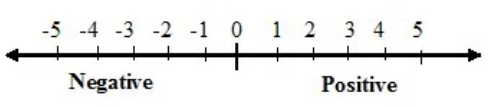## C++ Program to check whether a number is positive or negative

In this program we will find whether a number is positive, negative or zero series using if else statement. We would first declared and initialized the required variables. Next, we would prompt user to input any number. Later we will find that number is positive,negative or zero.

## Output

Positive Value.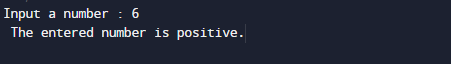Negative Value.Zero Value.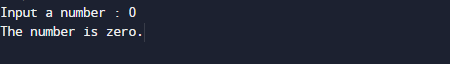In the above program, we have first declared and initialized a set variables required in the program.

• num = it will hold the value of number.

In the next statement user will be prompt to enter any number terms which will be assigned to variable ‘num’.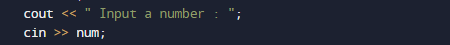For finding given integer is positive ,negative or zero we will use We will use nested if else statement.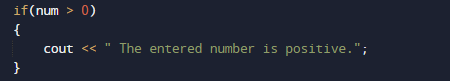in above image within the  if condition we check  if(num > 0), number is greater than zero then number is Positive.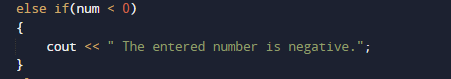if the given number is less then Zero else if(num < 0) then number is negative.

else the number is zero.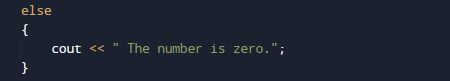In this program we  find that  a number is positive, negative or zero.The above approach is easiest way find the problem. First we check condition for positive number, then for negative or and later we find for zero.

In this tutorial we have learn about the C++ Program to check number positive or negative and its application with practical example. I hope you will like this tutorial.